# AnalyticBridge

A Data Science Central Community

In today’s blog post, we shall look into time series analysis using R package – forecast. Objective of the post will be explaining the different methods available in forecast package which can be applied while dealing with time series analysis/forecasting.

### What is Time Series?

A time series is a collection of observations of well-defined data items obtained through repeated measurements over time. For example, measuring the value of retail sales each month of the year would comprise a time series.

#### Objective:

• Identify patterns in the data – stationarity/non-stationarity.
• Prediction from previous patterns.

### Time series Analysis in R:

My data set contains data of Sales of CARS from Jan-2008 to Dec 2013.

#### Problem Statement:Forecast sales for 2013

MyData[1,1:14]

 PART Jan08 FEB08 MAR08 .... .... NOV12 DEC12 MERC 100 127 56 .... .... 776 557

Table: shows the first row data from Jan 2008 to Dec 2012

Results:

The forecasts of the timeseries data will be: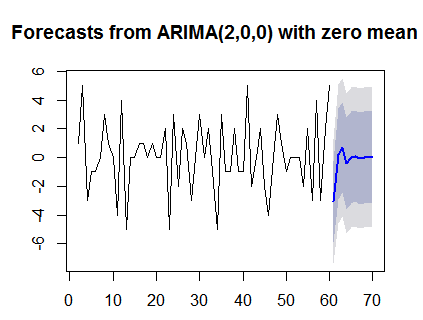Assuming that the data sources for the analysis are finalized and cleansing of the data is done, for further details,

#### Step1: Understand the data:

As a first step, Understand the data visually, for this purpose, the data is converted to time series object using ts(), and plotted visually using plot() functions available in R.

ts = ts(t(data[,7:66]))

plot(ts[1,],type=’o’,col=’blue’)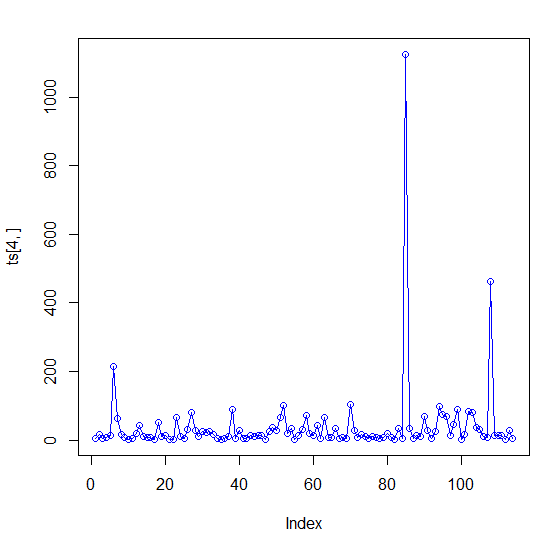Image above shows the monthly sales of an automobile

#### Forecast package & methods:

Forecast package is written by Rob J Hyndman and is available from CRAN here. The package contains Methods and tools for displaying and analyzing univariate time series forecasts including exponential smoothing via state space models and automatic ARIMA modelling.

Before going into more accurate Forecasting functions for Time series, let us do some basic forecasts using Meanf(), naïve(), random walk with drift – rwf() methods. Though these may not give us proper results but we can use the results as bench marks.

All these forecasting models returns objects which contain original series, point forecasts, forecasting methods used residuals. Below functions shows three methods & their plots.

Library(forecast)

mf = meanf(ts[,1],h=12,level=c(90,95),fan=FALSE,lambda=NULL)

plot(mf)mn = naive(ts[,1],h=12,level=c(90,95),fan=FALSE,lambda=NULL)

plot(mn)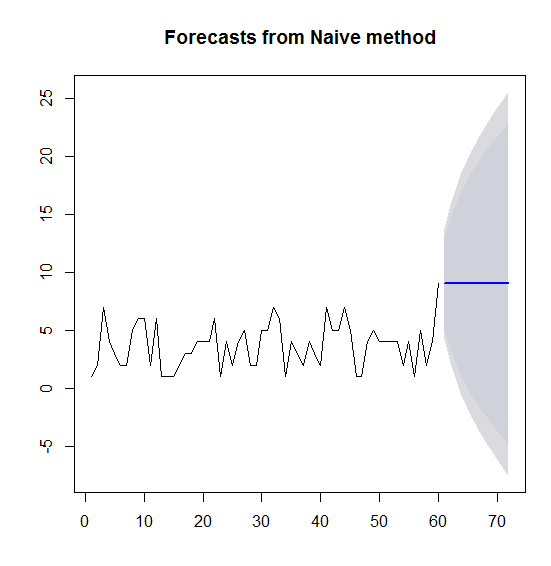md = rwf(ts[,1],h=12,drift=T,level=c(90,95),fan=FALSE,lambda=NULL)

plot(md)#### Measuring accuracy:

Once the model has been generated the accuracy of the model can tested using accuracy(). The Accuracy function returns MASE value which can be used to measure the accuracy of the model. The best model is chosen from the below results which gives have relatively lesser values of ME,RMSE,MAE,MPE,MAPE,MASE.

> accuracy(md)

ME     RMSE       MAE          MPE    MAPE     MASE

Training set      1.806244e-16 2.445734 1.889687 -41.68388 79.67588 1.197689

accuracy(mf)

ME      RMSE        MAE         MPE     MAPE MASE

Training set        1.55489e-16  1.903214 1.577778 -45.03219 72.00485         1

> accuracy(mn)

ME   RMSE       MAE         MPE      MAPE     MASE

Training set 0.1355932 2.44949 1.864407 -36.45951 76.98682 1.181666

#### Step2: Time Series Analysis Approach:

A typical time-series analysis involves below steps:

• Check for identifying under lying patterns - Stationary & non-stationary, seasonality, trend.
• After the patterns have been identified, if needed apply Transformations to the data – based on Seasonality/trends appeared in the data.
• Apply forecast() the future values using Proper ARIMA model obtained by auto.arima() methods.

#### Identify Stationarity/Non-Stationarity:

A stationary time series is one whose properties do not depend on the time at which the series is observed. Time series with trends, or with seasonality, are not stationary.

The stationarity /non-stationarity of the data can be known by applying Unit Root Tests - augmented Dickey–Fuller test (ADF), Kwiatkowski-Phillips-Schmidt-Shin (KPSS) test.

ADF: The null-hypothesis for an ADF test is that the data are non-stationary. So large p-values are indicative of non-stationarity, and small p-values suggest stationarity. Using the usual 5% threshold, differencing is required if the p-value is greater than 0.05.

library(tseries)

Augmented Dickey-Fuller Test

data:  ts[, 1]

Dickey-Fuller = -4.8228, Lag order = 3, p-value = 0.01

alternative hypothesis: stationaryThe above figure suggests us that the data is of stationary and we can go ahead with ARIMA models.

KPSS: Another popular unit root test is the Kwiatkowski-Phillips-Schmidt-Shin (KPSS) test. This reverses the hypotheses, so the null-hypothesis is that the data are stationary. In this case, small p-values (e.g., less than 0.05) suggest that differencing is required.

kpss = kpss.test(ts[,1])

Warning message:

In kpss.test(ts[, 1]) : p-value greater than printed p-value

kpss

KPSS Test for Level Stationarity

data:  ts[, 1]

KPSS Level = 0.1399, Truncation lag parameter = 1, p-value = 0.1

Differencing:

Based on the unit test results we identify whether the data is stationary or not. If the data is stationary then we choose optimal ARIMA models and forecasts the future intervals. If the data is non- stationary, then we use Differencing - computing the differences between consecutive observations. Use ndiffs(),diff() functions to find the number of times differencing needed for the data &  to difference the data respectively.

ndiffs(ts[,1])

 1

diff_data = diff(ts[,1])

Time Series:

Start = 2

End = 60

Frequency = 1

  1  5 -3 -1 -1  0  3  1  0 -4  4 -5  0  0  1  1  0  1  0  0  2 -5  3 -2  2  1 -3  0  3  0  2 -1 -5  3 -1

 -1  2 -1 -1  5 -2  0  2 -2 -4  0  3  1 -1  0  0  0 -2  2 -3  4 -3  2  5

Now retest for stationarity by applying acf()/kpss() functions if the plots shows us the Stationarity then Go ahead by applying ARIMA Models.

Identify Seasonality/Trend:

The seasonality in the data can be obtained by the stl()when plotted

Stl = Stl(ts[,1],s.window=”periodic”)

Series is not period or has less than two periods

Since my data doesn’t contain any seasonal behavior I will not touch the Seasonality part.

ARIMA Models:

For forecasting stationary time series data we need to choose an optimal ARIMA model (p,d,q). For this we can use auto.arima() function which can choose optimal (p,d,q) value and return us. Know more about ARIMA from here.

auto.arima(ts[,2])

Series: ts[, 2]

ARIMA(3,1,1) with drift

Coefficients:

ar1      ar2      ar3      ma1   drift

-0.2621  -0.1223  -0.2324  -0.7825  0.2806

s.e.   0.2264   0.2234   0.1798   0.2333  0.1316

sigma^2 estimated as 41.64:  log likelihood=-190.85

AIC=393.7   AICc=395.31   BIC=406.16

Forecast time series:

Now we use forecast() method to forecast the future events.

forecast(auto.arima(dif_data))   Point Forecast     Lo 80      Hi 80     Lo 95    Hi 9561   -3.076531531 -5.889584 -0.2634795 -7.378723 1.22566062    0.231773625 -2.924279  3.3878266 -4.594993 5.05854063    0.702386360 -2.453745  3.8585175 -4.124500 5.52927264   -0.419069906 -3.599551  2.7614107 -5.283195 4.44505565    0.025888991 -3.160496  3.2122736 -4.847266 4.89904466    0.098565814 -3.087825  3.2849562 -4.774598 4.97172967   -0.057038778 -3.243900  3.1298229 -4.930923 4.81684668    0.002733053 -3.184237  3.1897028 -4.871317 4.87678369    0.013817766 -3.173152  3.2007878 -4.860232 4.88786870   -0.007757195 -3.194736  3.1792219 -4.881821 4.866307

plot(forecast(auto.arima(dif_data)))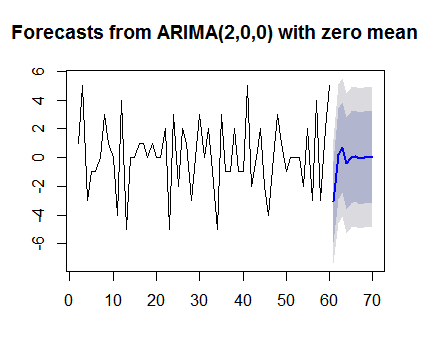The below flow chart will give us a summary of the time series ARIMA models approach: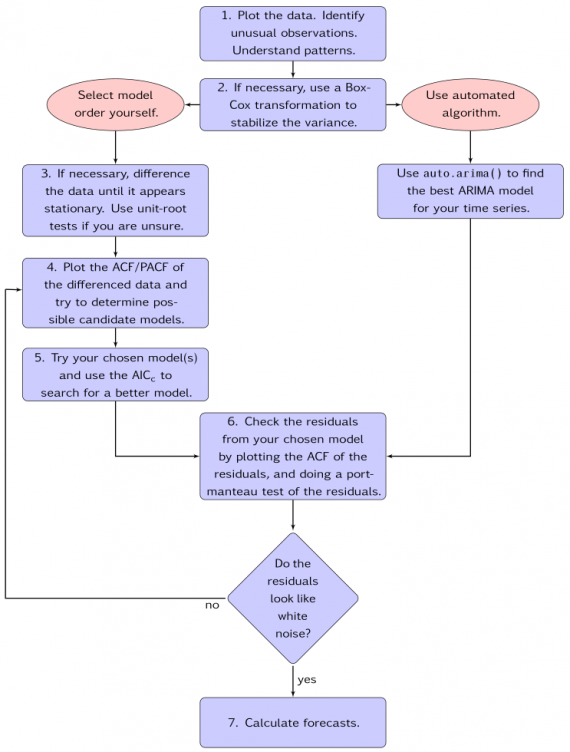The above flow diagram explains the steps to be followed for a time series forecasting

Please visit my blog dataperspective for more articles

Views: 23466

Tags: R, series, time

Comment

Join AnalyticBridgeComment by Ayu Sri lestari on February 8, 2018 at 6:20pm

hi, i have data with the same structure as above, but for multiple objects (let's say the objects are customer's id and i have 200K customer's id). do you have any idea how to solve that kind of data in R? *sorry for my bad english* thank youComment by Norberto J. Sanchez on September 3, 2017 at 10:42am

I like your post. However, it's hard to follow or replicate your method without a reference or access to the car sales data set. Am I missing something?Comment by Biswarup Ghosh on December 16, 2015 at 3:38pm

this post is a blatant copy from Rob J Hyndman's book .Atleast acknowledge the source